Mathematical Logic as based on the Theory of Types

BY   BERTRAND  RUSSELL

Published in: American Journal of Mathematics, vol.30(1908), pp. 222-262
Transcribed into hypertext by Burtzev B.I., Jul., 07, 2003
e-mail: bbi-math@narod.ru,   site: http://bbi-math.narod.ru/

X.

Ordinal Numbers.

FK.An ordinal number is a class of ordinally similar well-ordered series, i.e., of relations generating such series. Ordinal similarity or likeness is defined as follows:

Smor = P^ Q^ { (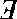S) . S ε1 --> 1 . Dinv*S = C*Q . P = S|Q|Sº  Df.,

where УSmorФ is short for Уsimilar ordinally.Ф
FL.The class of serial relations, which we will call УSer,Ф is defined as follows:

Ser = P^ { xPy .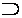x, y . ~( x = y) : xPy . yPz .x, y, z . xPz :

x ε C*P .x .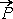*x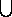ι*x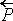*x = C*P }   Df.
FM.That is, reading P as is Уprecedes,Ф a relation is serial if (l) no term precedes itself, (2) a predecessor of a predecessor is a predecessor, (3) if x is any term in the field of the relation, then the predecessors of x together with x together with the successors of x constitute the whole field of the relation.
FN.Well-ordered serial relations, which we will call Ω, are defined as follows:

Ω = P^ { P ε Ser: α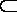C*P .! α .α .! ( α - Pº**α).}  Df;

i.e., P generates a well-ordered series if P is serial, and any class α contained in the field of P and not null has a first term. (Note that Pº**α are the terms coming after some term of α).
FO.If we denote by No*P the ordinal number of a well-ordered relation P, and by NO the class of ordinal numbers, we shall have
 No = α^ P^ { P ε Ω . α = Smor----->*P }  Df. NO = No**Ω.
From the definition of No we have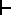: P ε Ω .. No*P = Smor----->*P: ~( P ε Ω) . ~E ! . No*P.
FP.If we now examine our definitions with a view to their connection with the theory of types, we see, to begin with, that the definitions of УSerФ and Ω involve the fields of serial relations. Now the field is only significant when the relation is homogeneous; hence relations which are not homogeneous do not generate series. For example, the relation ι might be thought to generate series of ordinal number ω, such as

x,    ι*x,    ι*ι*x, . . . . . ιn*x,. . . . . ,

and we might attempt to prove in this way the existence of ω and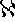0. But x and ι*x are of different types, and therefore there is no such series according to the definition.
FQ.The ordinal number of a series of individuals is, by the above definition of No, a class of relations of individuals. It is therefore of a different type from any individual, and can not form part of any series in which individuals occur. Again, suppose all the finite ordinals exist as individual ordinals; i.e., as the ordinals of series of individuals. Then the finite ordinals themselves form a series whose ordinal number is ω; thus ω exists as an ordinal-ordinal, i.e., as the ordinal of a series of ordinals. But the type of an ordinal-ordinal is that of classes of relations of classes of relations of individuals. Thus the existence of ω has been proved in a higher type than that of the finite ordinals. Again, the cardinal number of ordinal numbers of well-ordered series that can be made out of finite ordinals is1; hence1 exists in the type of classes of classes of classes of relations of classes of relations of individuals. Also the ordinal numbers of well-ordered series composed of finite ordinals can be arranged in order of magnitude, and the result is a well-ordered series whose ordinal number is ω1. Hence ω1 exists as an ordinal-ordinal-ordinal. This process can be repeated any finite number of times, and thus we can establish the existence, in appropriate types, ofn and ωn for any finite value of n.
FR.But the above process of generation no longer leads to any totality of all ordinals, because, if we take all the ordinals of any given type, there are always greater ordinals in higher types; and we can not add together a set of ordinals of which the type rises above any finite limit. Thus all the ordinals in any type can be arranged by order of magnitude in a well-ordered series, which has an ordinal number of higher type than that of the ordinals composing the series. In the new type, this new ordinal is not the greatest. In fact, there is no greatest ordinal in any type, but in every type all ordinals are less than some ordinals of higher type. It is impossible to complete the series of ordinals, since it rises to types above every assignable finite limit; thus although every segment of the series of ordinals is well-ordered, we can not say that the whole series is well-ordered, because the Уwhole seriesФ is a fiction. Hence Burali-FortFs contradiction disappears.
FS.From the last two sections it appears that, if it is allowed that the number of individuals is not finite, the existence of all CantorТs cardinal and ordinal numbers can be proved, short ofω and ωω. (It is quite possible that the existence of these may also be demonstrable.) The existence of all finite cardinals and ordinals can be proved without assuming the existence of anything. For if the cardinal number of terms in any type is n, that of terms in the next type is 2n. Thus if there are no individuals, there will be one class (namely, the null-class), two classes of classes (namely, that containing no class and that containing the null-class), four classes of classes of classes, and generally 2n - 1 classes of the nth order. But we can not add together terms of different types, and thus we can not in this way prove the existence of any infinite class.
FT.We can now sum up our whole discussion. After stating some of the paradoxes of logic, we found that all of them arise from the fact that an expression referring to all of some collection may itself appear to denote one of the collection; as, for example, Уall propositions are either true or falseФ appears to be itself a proposition. We decided that, where this appears to occur, we are dealing with a false totality, and that in fact nothing whatever can significantly be said about all of the supposed collection. In order to give effect to this decision, we explained a doctrine of types of variables, proceeding upon the principle that any expression which refers to all of some type must, if it denotes anything, denote something of a higher type than that to all of which it refers. Where all of some type is referred to, there is an apparent variable belonging to that type. Thus any expression containing an apparent variable is of higher type than that variable. This is the fundamental principle of the doctrine of types. A change in the manner in which the types are constructed, should it prove necessary, would leave the solution of contradictionsuntouched so long as this fundamental principle is observed. The method of constructing types explained above was shown to enable us to state all the fundamental definitions of mathematics, and at the same time to avoid all known contradictions. And it appeared that in practice the doctrine of types is never relevant except where existence-theorems are concerned, or where applications are to be made to some particular case.
FU.The theory of types raises a number of difficult philosophical questions concerning its interpretation. Such questions are, however, essentially separable from the mathematical development of the theory, and, like all philosophical questions, introduce elements of uncertainty which do not belong to the theory itself. It seemed better, therefore, to state the theory without reference to philosophical questions, leaving these to be dealt with independently.